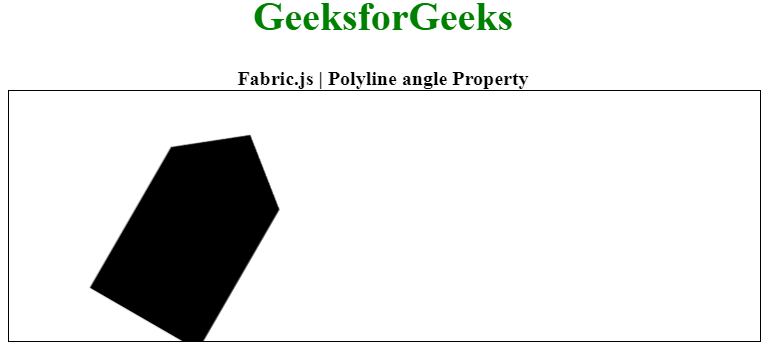Related Articles
Fabric.js Polyline angle Property
• Last Updated : 20 Jan, 2021

In this article, we are going to see how to set the angle of rotation of an object in the degree of a canvas Polyline using FabricJS. The canvas Polyline means Polyline is movable and can be stretched according to requirement. Further, the Polyline can be customized when it comes to initial stroke color, height, width, fill color, or stroke width.

To make it possible, we are going to use a JavaScript library called FabricJS. After importing the library using CDN, we will create a canvas block in the body tag that will contain our Polyline. After this, we will initialize instances of Canvas and Polyline provided by FabricJS and use the angle property to rotate the text to the required angle and render the Canvas on the Polyline as given in the example below.

Syntax:

```var polyline = new fabric.Polyline(Points, {
angle: number
});  ```

Parameters: This property accepts a single parameter as mentioned above and described below:

• angle: It specifies the angle of rotation.

The below example illustrates the angle property in Fabric.js:

Example:

## HTML

 ` ``<``html``> `` ` `<``head``> ``    ````    ``<``script` `src``= ``"https://cdnjs.cloudflare.com/ajax/libs/fabric.js/3.6.2/fabric.min.js"``> ``    `` `` `` ` `<``body``> ``    ``<``div` `style``=``"text-align: center;width: 600px;"``> ``      ``<``h1` `style``=``"color: green;"``> ``          ``GeeksforGeeks ``      `` `` ` `      ``<``b``> ``          ``Fabric.js | Polyline angle Property ``      `` ``    `` ``   ` `    ``<``canvas` `id``=``"canvas"``            ``width``=``"600"``            ``height``=``"200"``            ``style``=``"border:1px solid #000000;"``> ``    `` `` ` `    ``<``script``> `` ` `        ``// Initiate a Canvas instance ``        ``var canvas = new fabric.Canvas("canvas"); `` ` `        ``// Initiate a polyline instance ``        ``var polyline = new fabric.Polyline([ ``        ``{ x: 200, ``            ``y: 10 }, ``        ``{x: 250, ``            ``y: 50 ``        ``}, { ``            ``x: 250, ``            ``y: 180 ``        ``}, { ``            ``x: 150, ``            ``y: 180 ``        ``}, { ``            ``x: 150, ``            ``y: 50 ``        ``}, { ``            ``x: 200, ``            ``y: 10 }], { ``            ``angle: 30 ``        ``}); `` ` `        ``// Render the polyline in canvas ``        ``canvas.add(polyline); ``    `` `` `` ` ``

Output:My Personal Notes arrow_drop_up